## Model and Simulate CubeSats

To create models, use the CubeSat Vehicle blocks, model template, and project. Explore the spacecraft example modeling multiple spacecraft. The CubeSat Vehicle block propagates one satellite at a time. To propagate multiple satellites simultaneously, use the Orbit Propagator block. To calculate shortest quaternion rotation, use the Attitude Profile block.

To help you get started modeling and simulating spacecraft, Aerospace Blockset™ provides a project and model on the Simulink® Start Page.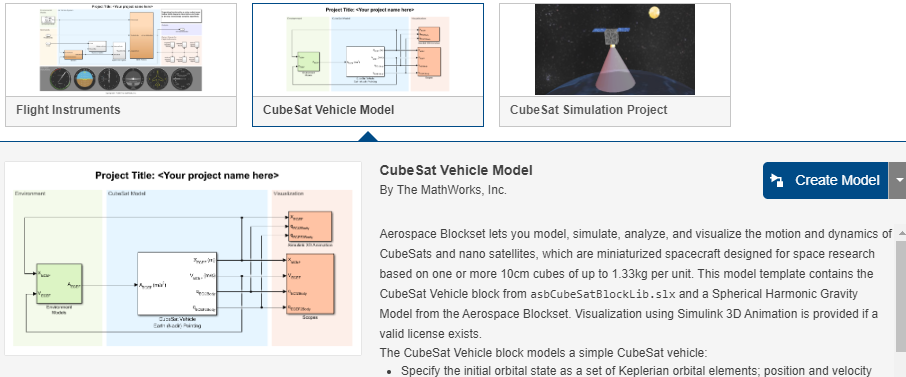• CubeSat Vehicle Model template — A model template (```CubeSat Simulation Project```) that illustrates how to propagate and visualize CubeSat trajectories using the CubeSat Vehicle block. The Spherical Harmonic Gravity Model block is used as the gravitational potential source for orbit propagation. The preconfigured pointing modes set in the CubeSat Vehicle block control the attitude.

• CubeSat Simulation Project — A ready-to-simulate project (```CubeSat Simulation Project```) that illustrates how to create a detailed CubeSat system design in Simulink by adding in detailed vehicle components to the provided framework.

### CubeSat Vehicle Model Template

The template model is a ready-to-simulate example that contains a CubeSat Vehicle block with visualization using Simulink 3D Animation™.

1. Start the CubeSat Vehicle Model template.2. Click Create Model.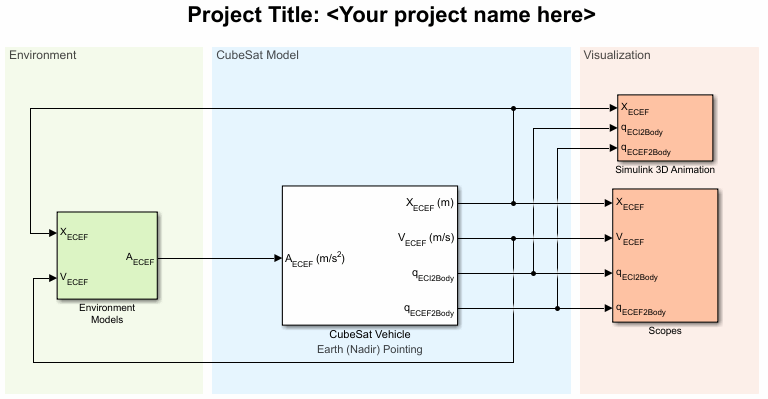3. The CubeSat Vehicle block models a simple CubeSat vehicle that you can use as is, with the CubeSat Vehicle block configured to use the initial orbital state as a set of Keplerian orbital elements.

The model uses the Spherical Harmonic Gravity Model block to provide the vehicle gravity for the CubeSat.

To familiarize yourself with CubeSats, experiment with the CubeSat Vehicle block settings.

• On the CubeSat Orbit tab of the block, you can optionally use the Input method parameter to change the initial orbital state as a set of:

• Position and velocity state vectors in Earth-centered inertial axes

• Position and velocity state vectors in Earth-centered Earth-fixed axes

• Latitude, longitude, altitude, and velocity of the body with respect to ECEF, expressed in the NED frame

• On the CubeSat Attitude tab, you can specify the alignment and constraint vectors to define the CubeSat attitude control.

• The CubeSat vehicle first aligns the primary alignment vector with the primary constraint vector. The CubeSat vehicle then attempts to align the secondary alignment vector with the secondary constraint vector as closely as possible without affecting primary alignment.

• The CubeSat Altitude tab also lets you choose between preconfigured Earth (Nadir) Earth Pointing (default) and Sun Tracking attitude control modes.

• On the Earth Orientation Parameters tab, you can direct the block to include higher order earth orientation parameters in transformations between the ECI and ECEF coordinate systems.

4. Run and simulate the model.

5. To view the output signals from the CubeSat, double-click the Scopes subsystem and open the multiple scopes.

6. If you have a valid Simulink 3D Animation license, you can also visualize the orbit in the CubeSat Orbit Animation window.

7. Save a copy of the orbit propagation model. You can use this model for the mission analysis live script.

The CubeSat Vehicle Model template CubeSat Vehicle block uses simple preconfigured orbit and attitude control modes. To model and simulate CubeSat vehicles using your own detailed components, consider the CubeSat Simulation Project from the Simulink Start Page. For more information, see CubeSat Simulation Project

### CubeSat Simulation Project

The model is a ready-to-simulate example with visualization using Simulink 3D Animation. This example uses a Vehicle Model subsystem in place of a CubeSat Vehicle block. For a simpler model that illustrates the use of the CubeSat Vehicle block, see CubeSat Vehicle Model Template.

1. Start the CubeSat Simulation Project.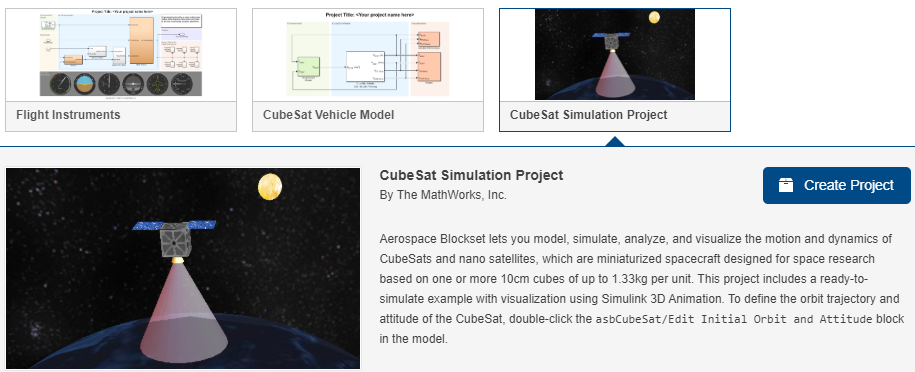2. Click Create Project and follow the instructions.3. The Vehicle Model subsystem models a CubeSat vehicle that you can use as is.

To create your own more sophisticated satellite models, experiment with the Vehicle Model framework For example, you can replace the perfect thruster model included by default in the actuator subsystem with your own more realistic thruster or reaction wheel model.

4. To change the orbit trajectory and attitude of the CubeSat, in the Mission Configuration section, double-click the Edit Initial Orbit and Attitude block. These parameters have the same intent as the corresponding parameters as the CubeSat Vehicle block.

5. Run and simulate the model.

6. To view the output signals from the CubeSat, double-click the Scopes subsystem and open the multiple scopes.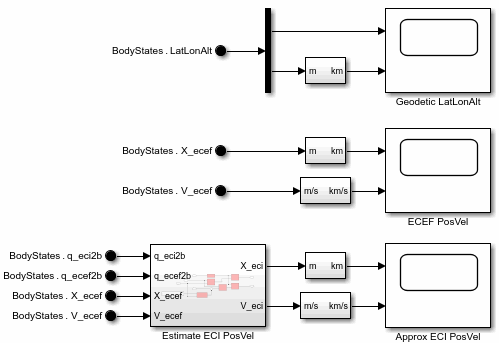7. If you have a license for Simulink 3D Animation, you can also visualize the orbit in an animation window. Double-click the Visualization subsystem and click the Open Simulink 3D Animation window button.

The CubeSat Orbit Animation window opens.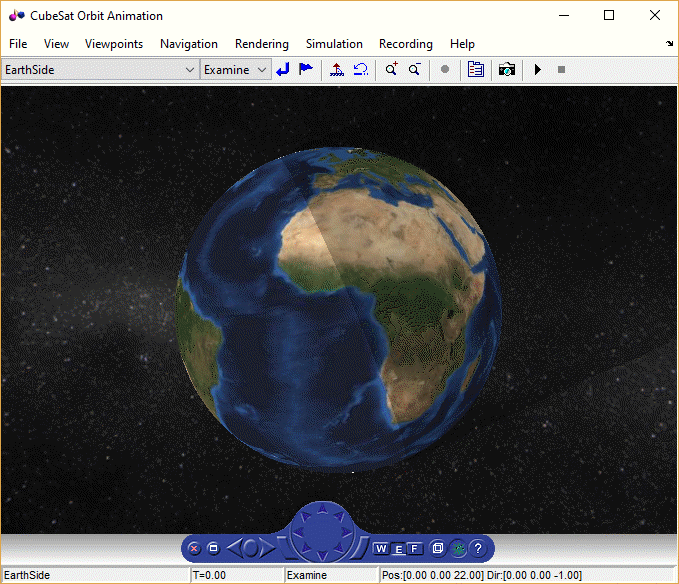### Utility Functions

Aerospace Toolbox provides utility functions for coordinate transformations. You can use these functions to go between the various initial condition modes of the CubeSat Vehicle block.

ActionFunction

Calculate position and velocity vectors in Earth-centered inertial mean-equator mean-equinox

`ecef2eci`

Calculate position, velocity, and acceleration vectors in Earth-centered Earth-fixed (ECEF) coordinate system

`eci2ecef`

Calculate Greenwich mean and apparent sidereal times

`siderealTime`

Calculate Keplerian orbit elements using geocentric equatorial position and velocity vectors

`ijk2keplerian`

Calculate position and velocity vectors in geocentric equatorial coordinate system using Keplerian orbit elements

`keplerian2ijk`

 Vallado, D. A. Fundamentals of Astrodynamics and Applications. New York: McGraw-Hill, 1997.

## SupportGet trial now